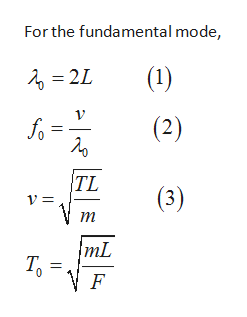# A taut string is tied down at both ends. Assume that the fundamental mode of oscillation of the string has a period T0. For each of the changes described below give the factor by which the period changes. For example, if the change described resulted in a period twice as long, you would put the number "2". Do not cumulate changes. That is, before each change, assume you are back at the original starting situation. (If your answer is not an integer, you can round it off to two sigfigs.)A. The string is replaced by one of twice the mass but of the same length. _____B. The wavelength of the starting shape is divided by three. __1/3__C. The amplitude of the oscillation is doubled. ______D. The tension of the string is halved. ______

Question
48 views

A taut string is tied down at both ends. Assume that the fundamental mode of oscillation of the string has a period T0. For each of the changes described below give the factor by which the period changes. For example, if the change described resulted in a period twice as long, you would put the number "2". Do not cumulate changes. That is, before each change, assume you are back at the original starting situation. (If your answer is not an integer, you can round it off to two sigfigs.)

• A. The string is replaced by one of twice the mass but of the same length. _____
• B. The wavelength of the starting shape is divided by three. __1/3__
• C. The amplitude of the oscillation is doubled. ______
• D. The tension of the string is halved. ______
check_circle

Step 1

the relation between velocity and wavelength will behelp_outlineImage TranscriptioncloseFor the fundamental mode, (1) 2L (2) fo TL (3) т mL Т, F fullscreen
Step 2

For the first and ...

### Want to see the full answer?

See Solution

#### Want to see this answer and more?

Solutions are written by subject experts who are available 24/7. Questions are typically answered within 1 hour.*

See Solution
*Response times may vary by subject and question.
Tagged in

### Wave Motion